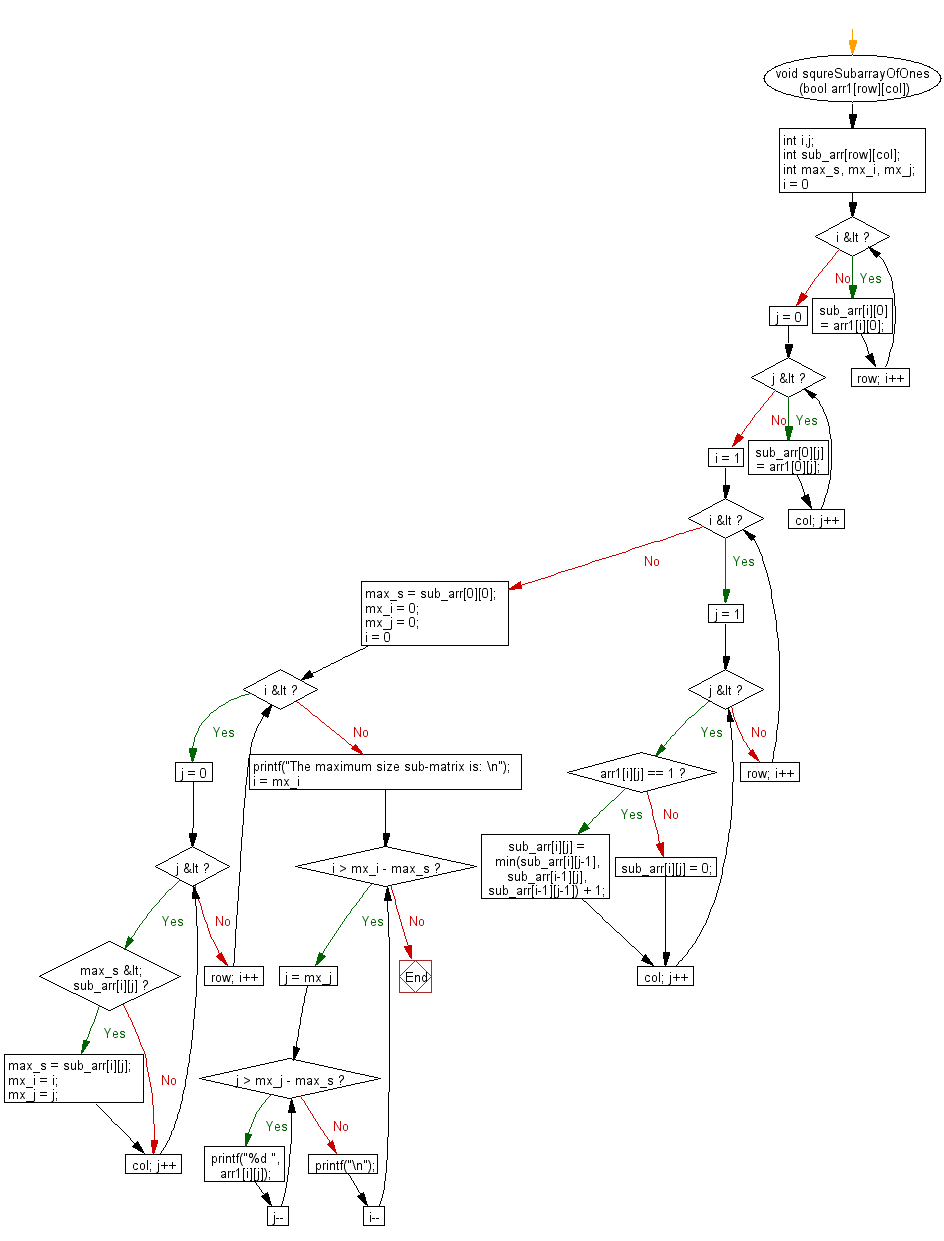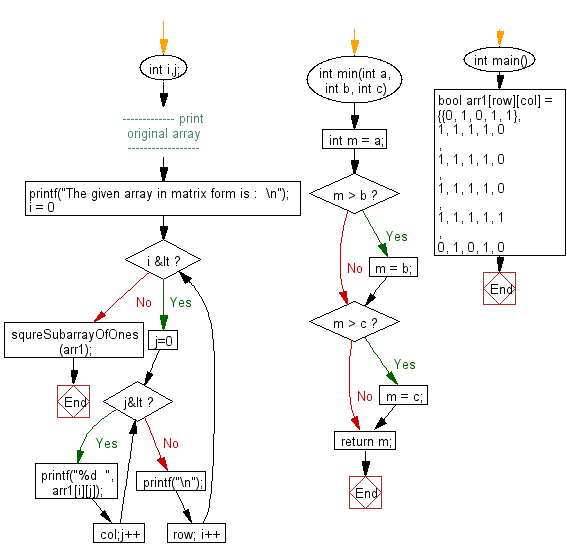﻿ C exercises: Find maximum size square sub-matrix with all 1s - w3resource# C Exercises: Find maximum size square sub-matrix with all 1s

## C Array: Exercise-89 with Solution

Write a program in C to find maximum size square sub-matrix with all 1s.

Sample Solution:

C Code:

``````// C/C++ code for Maximum size square sub-matrix with all 1s
#include<stdio.h>
#define bool int
#define row 6
#define col 5

void squreSubarrayOfOnes (bool arr1[row][col])
{
int i,j;
int sub_arr[row][col];
int max_s, mx_i, mx_j;

for(i = 0; i < row; i++)
sub_arr[i] = arr1[i];

for(j = 0; j < col; j++)
sub_arr[j] = arr1[j];

for(i = 1; i < row; i++)
{
for(j = 1; j < col; j++)
{
if(arr1[i][j] == 1)
sub_arr[i][j] = min(sub_arr[i][j-1], sub_arr[i-1][j], sub_arr[i-1][j-1]) + 1;
else
sub_arr[i][j] = 0;
}
}

max_s = sub_arr; mx_i = 0; mx_j = 0;
for(i = 0; i < row; i++)
{
for(j = 0; j < col; j++)
{
if(max_s < sub_arr[i][j])
{
max_s = sub_arr[i][j];
mx_i = i;
mx_j = j;
}
}
}

printf("The maximum size sub-matrix is: \n");
for(i = mx_i; i > mx_i - max_s; i--)
{
for(j = mx_j; j > mx_j - max_s; j--)
{
printf("%d ", arr1[i][j]);
}
printf("\n");
}
}

int min(int a, int b, int c)
{
int m = a;
if (m > b)
m = b;
if (m > c)
m = c;
return m;
}

int main()
{
bool arr1[row][col] =  {{0, 1, 0, 1, 1},
{1, 1, 1, 1, 0},
{1, 1, 1, 1, 0},
{1, 1, 1, 1, 0},
{1, 1, 1, 1, 1},
{0, 1, 0, 1, 0}};
int i,j;
//------------- print original array ------------------
printf("The given array in matrix form is :  \n");
for(i = 0; i < row; i++)
{
for (j=0;j<col;j++)
{
printf("%d  ", arr1[i][j]);
}
printf("\n");
}
//------------------------------------------------------

squreSubarrayOfOnes(arr1);
}
```
```

Sample Output:

```The given array in matrix form is :
0  1  0  1  1
1  1  1  1  0
1  1  1  1  0
1  1  1  1  0
1  1  1  1  1
0  1  0  1  0
The maximum size sub-matrix is:
1 1 1 1
1 1 1 1
1 1 1 1
1 1 1 1
```

Pictorial Presentation:Flowchart:C Programming Code Editor:

Improve this sample solution and post your code through Disqus.

﻿

## C Programming: Tips of the Day

Where is the C auto keyword used?

auto is a modifier like static. It defines the storage class of a variable. However, since the default for local variables is auto, you don't normally need to manually specify it.

Ref : https://bit.ly/3yzwC9r